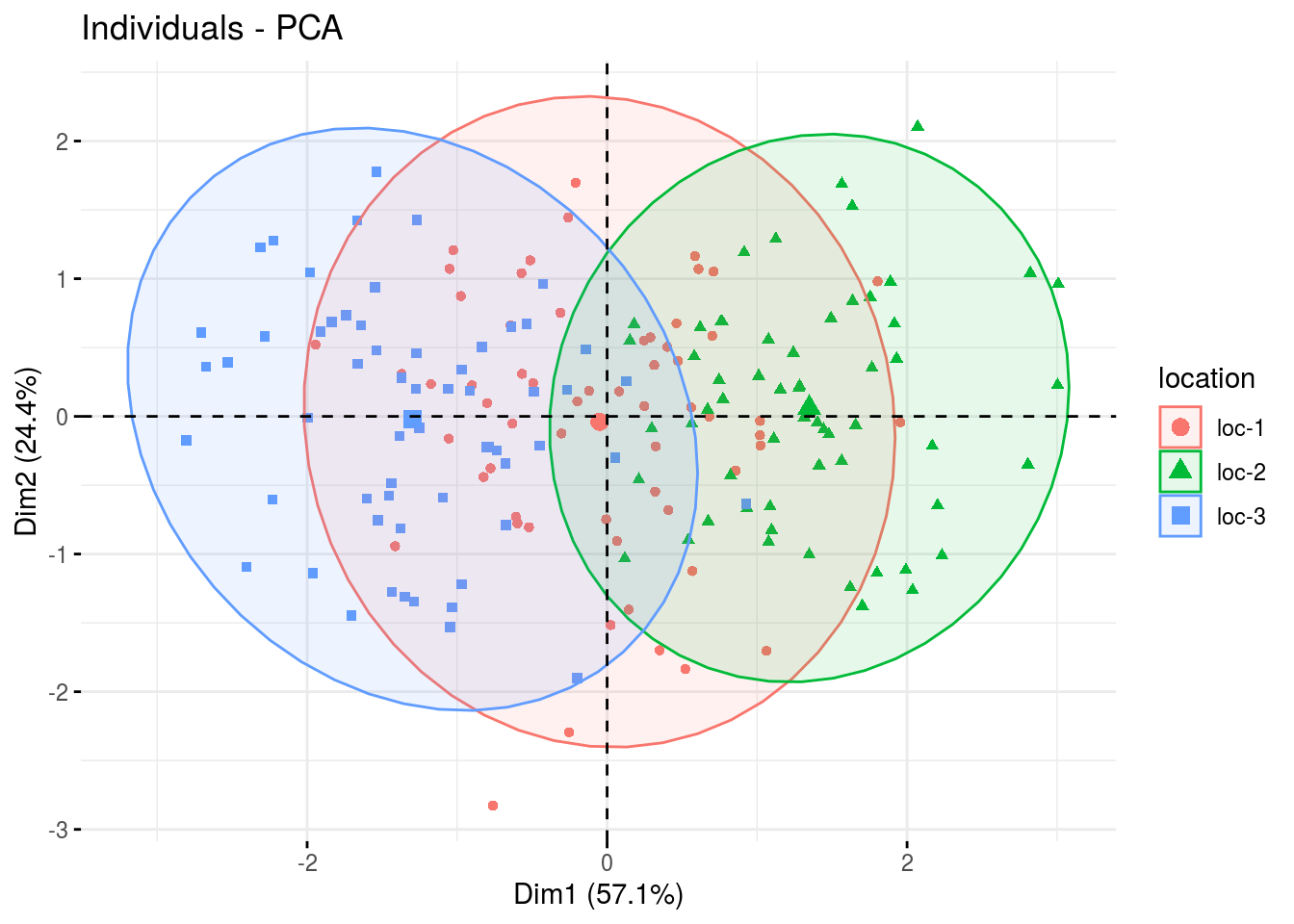## 3.9 Family 5 of analysis : multivariate analysis

Multivariate analysis can answer to multiple objective. One of them can be to study diversity structure and identify parents to cross based on either good complementarity or similarity for some traits

### 3.9.1 Decision tree

To study diversity structure and identify parents to cross based on either good complementarity or similarity for some traits, different scenario are possible (Figure 3.29). It can be completed by analysis of molecular data and genetic distance trees (M3, section 5).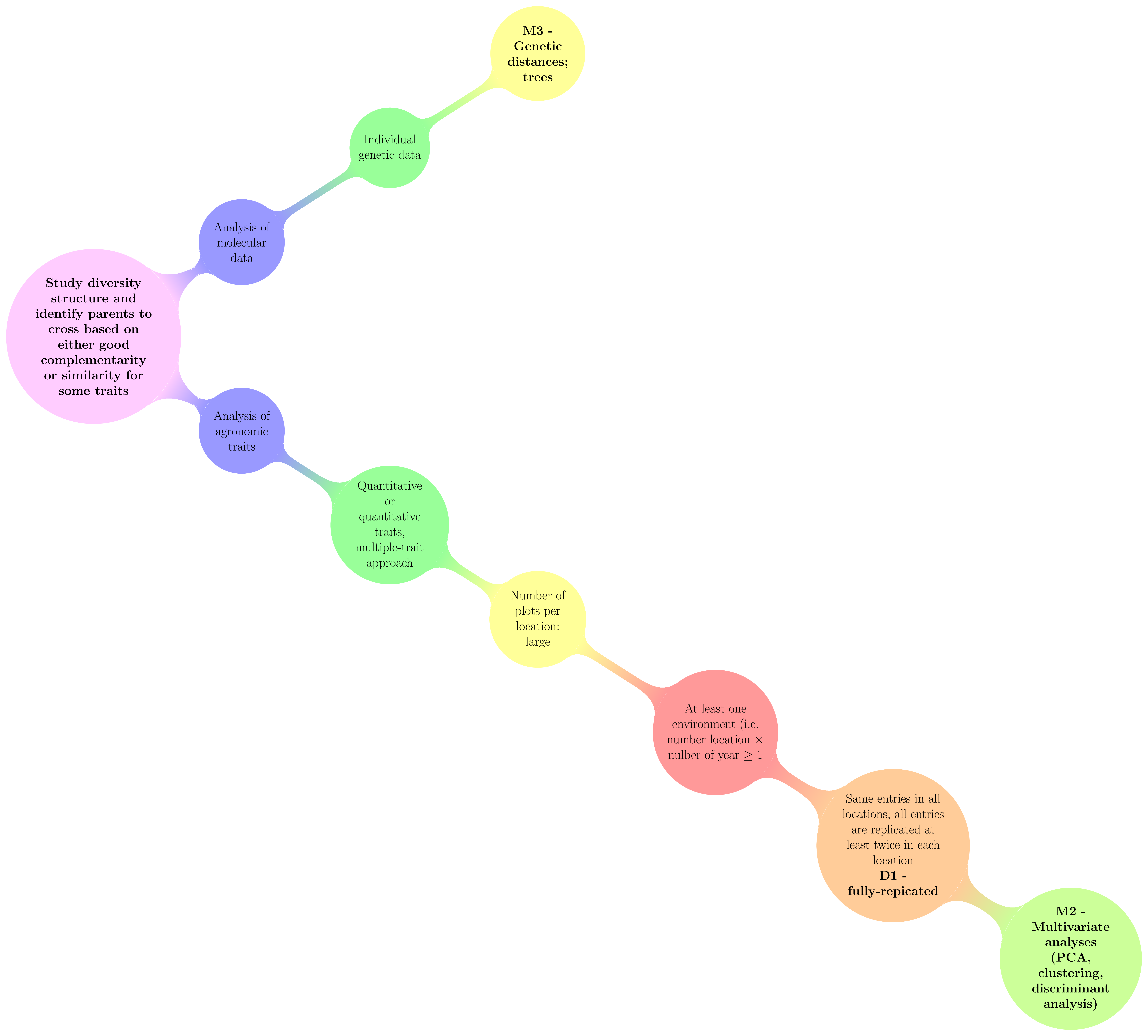Figure 3.29: Decision tree with experimental constraints, designs and methods of agronomic analysis carry out in PPBstats regarding the objective :Study diversity structure and identify parents to cross based on either good complementarity or similarity for some traits. D refers to designs and M to methods.

### 3.9.2 Workflow and function relations in PPBstats regarding family 2 of analysis

Figure 3.30 displays the functions and their relationships. Table 3.6 describes each of the main functions.

You can have more information for each function by typing ?function_name in your R session.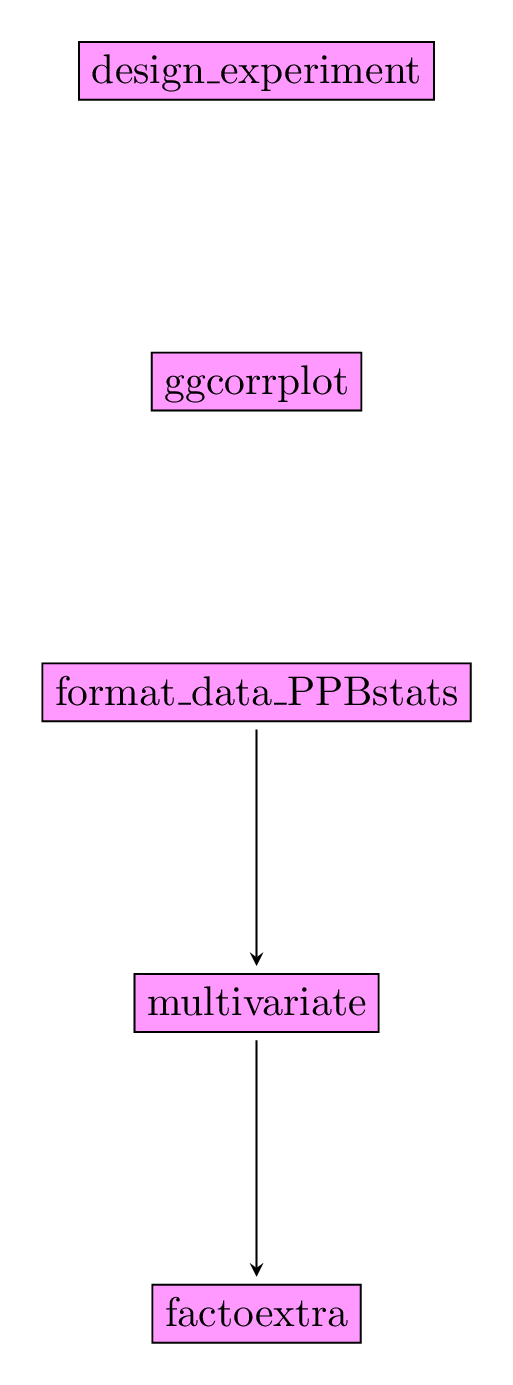Figure 3.30: Main functions used in the workflow of family 5.

Table 3.6: Function description.
function name description
design_experiment Provides experimental design for the different situations corresponding to the choosen family of analysis
format_data_PPBstats Check and format the data to be used in PPBstats functions
ggcorrplot Visualize correlation with ggcorrplot fonction from package ggcorrplot
multivariate Run multivariate analysis with functions from FactoMineR
factoextra Check outputs and results with the factoextra package

### 3.9.3 Steps with PPBstats

For variance intra analysis, you can follow these steps (Figure 3.2) :

• Format the data with format_data_PPBstats()
• Look at correlation between variables with ggcorrplot::ggcorrplot()12
• Run the analysis with multivariate()
• Check outputs and results with functions from factoextra13

### 3.9.4 Format the data

data("data_model_GxE")
data_model_GxE = format_data_PPBstats(data_model_GxE, type = "data_agro")
## data has been formated for PPBstats functions.

### 3.9.5 Look at correlation

library(ggcorrplot)
vec_variables = c("y1", "y2", "y3")
corr = round(cor(data_model_GxE[,vec_variables]), 1)
p.mat = cor_pmat(data_model_GxE[,vec_variables])

# Barring the no significant coefficient
ggcorrplot(corr, hc.order = TRUE, type = "lower", p.mat = p.mat)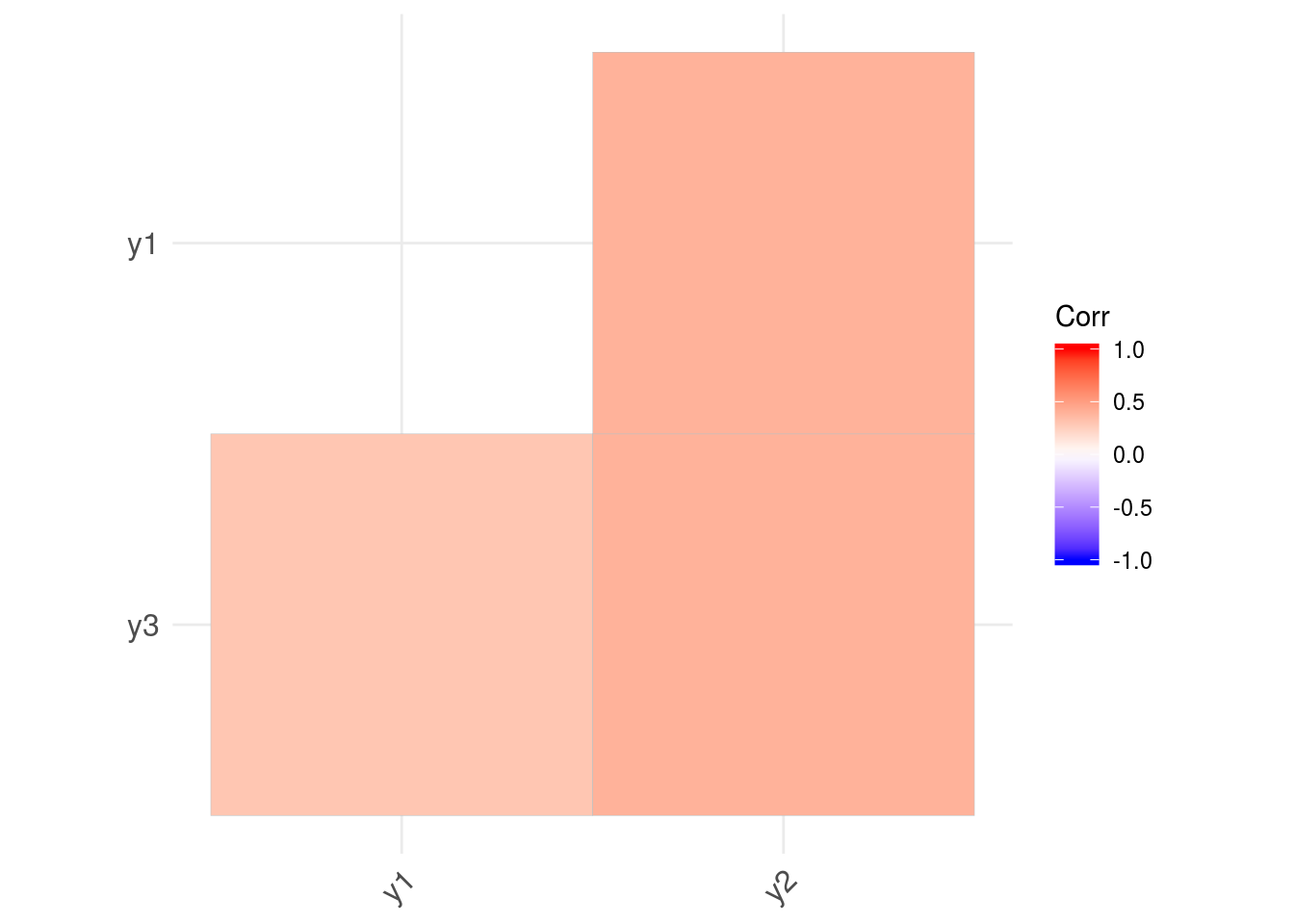More details on the use of ggcorrplot() can be found here.

### 3.9.6 Run the analysis

vec_variables = c("y1", "y2", "y3")
res.pca = mutlivariate(data_model_GxE, vec_variables, PCA)

### 3.9.7 Check outputs and results

Look at the results thanks to the factoextra package:

fviz_eig(res.pca)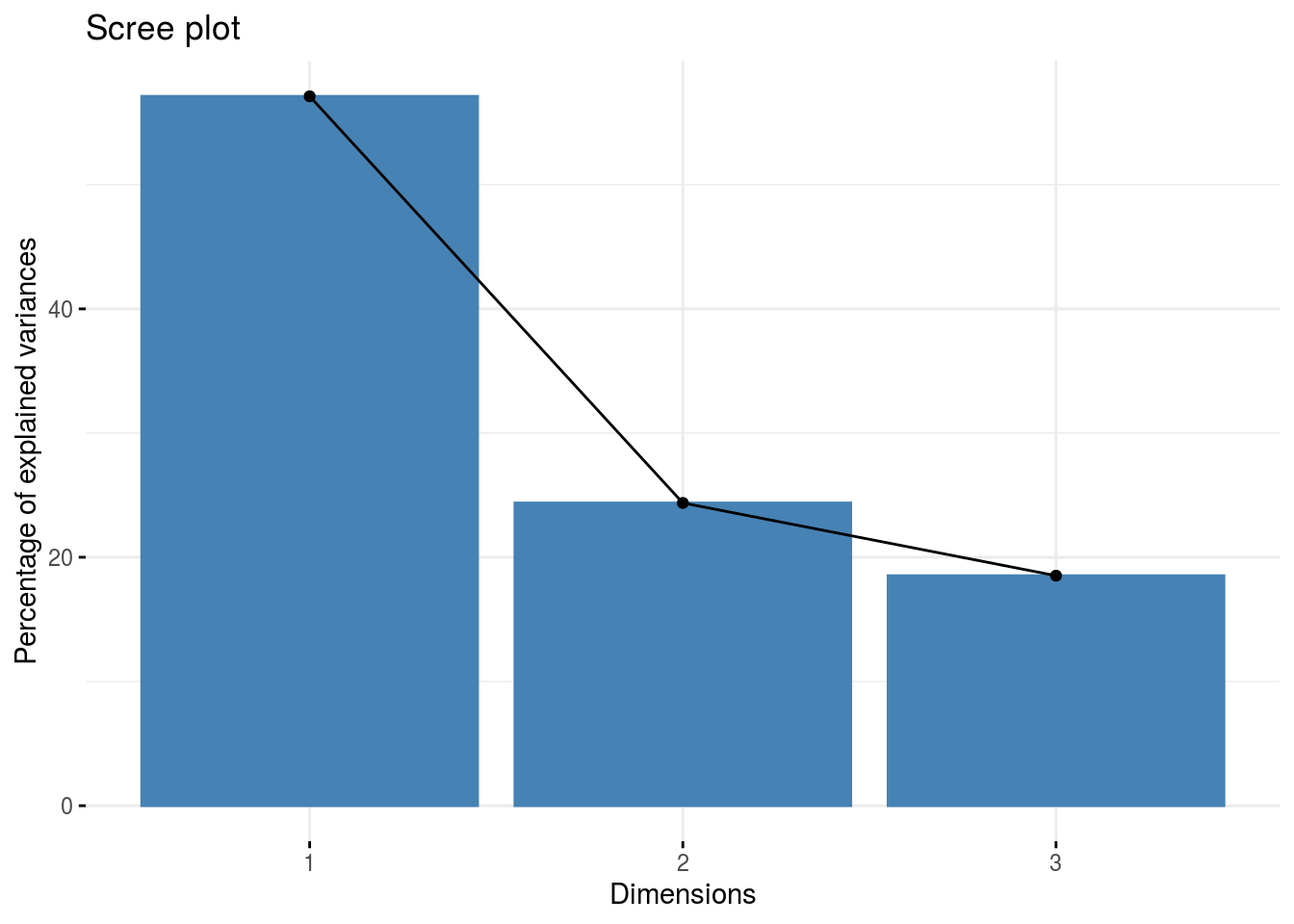fviz_pca_ind(res.pca, label="none", habillage="location", addEllipses=TRUE, ellipse.level=0.95)